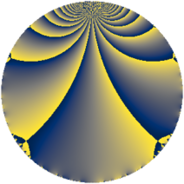# Properties

 Label 287.2.aLevel $287$ Weight $2$ Character orbit 287.a Rep. character $\chi_{287}(1,\cdot)$ Character field $\Q$ Dimension $21$ Newform subspaces $6$ Sturm bound $56$ Trace bound $3$

# Related objects

## Defining parameters

 Level: $$N$$ $$=$$ $$287 = 7 \cdot 41$$ Weight: $$k$$ $$=$$ $$2$$ Character orbit: $$[\chi]$$ $$=$$ 287.a (trivial) Character field: $$\Q$$ Newform subspaces: $$6$$ Sturm bound: $$56$$ Trace bound: $$3$$ Distinguishing $$T_p$$: $$2$$, $$3$$

## Dimensions

The following table gives the dimensions of various subspaces of $$M_{2}(\Gamma_0(287))$$.

Total New Old
Modular forms 30 21 9
Cusp forms 27 21 6
Eisenstein series 3 0 3

The following table gives the dimensions of the cuspidal new subspaces with specified eigenvalues for the Atkin-Lehner operators and the Fricke involution.

$$7$$$$41$$FrickeDim.
$$+$$$$+$$$$+$$$$2$$
$$+$$$$-$$$$-$$$$9$$
$$-$$$$+$$$$-$$$$8$$
$$-$$$$-$$$$+$$$$2$$
Plus space$$+$$$$4$$
Minus space$$-$$$$17$$

## Trace form

 $$21q + q^{2} + 17q^{4} + 2q^{5} + 4q^{6} - q^{7} + 9q^{8} + 25q^{9} + O(q^{10})$$ $$21q + q^{2} + 17q^{4} + 2q^{5} + 4q^{6} - q^{7} + 9q^{8} + 25q^{9} + 10q^{10} - 4q^{11} + 12q^{12} + 14q^{13} - 3q^{14} - 16q^{15} + 13q^{16} + 14q^{17} - 7q^{18} - 22q^{20} - 4q^{23} - 24q^{24} + 39q^{25} - 18q^{26} + 12q^{27} - 7q^{28} + 2q^{29} - 28q^{30} - 24q^{31} + 29q^{32} + 16q^{33} - 2q^{34} - 2q^{35} + 5q^{36} + 10q^{37} - 28q^{38} + 12q^{39} - 6q^{40} + q^{41} + 12q^{42} + 36q^{43} - 36q^{44} - 22q^{45} - 40q^{46} - 28q^{48} + 21q^{49} - 29q^{50} + 12q^{51} + 66q^{52} - 18q^{53} - 44q^{54} + 16q^{55} - 15q^{56} - 4q^{57} - 18q^{58} + 24q^{59} - 116q^{60} + 2q^{61} - 16q^{62} - 5q^{63} + q^{64} - 32q^{65} - 80q^{66} - 4q^{67} - 22q^{68} - 52q^{69} - 10q^{70} - 44q^{71} - 41q^{72} + 54q^{73} + 8q^{74} - 8q^{75} - 4q^{77} - 30q^{78} - 20q^{79} - 70q^{80} + 37q^{81} + 3q^{82} - 4q^{83} - 2q^{84} + 20q^{85} + 32q^{86} + 20q^{87} + 36q^{88} - 38q^{89} + 30q^{90} + 2q^{91} + 42q^{92} + 32q^{93} + 4q^{94} - 48q^{96} + 30q^{97} + q^{98} - 24q^{99} + O(q^{100})$$

## Decomposition of $$S_{2}^{\mathrm{new}}(\Gamma_0(287))$$ into newform subspaces

Label Dim. $$A$$ Field CM Traces A-L signs $q$-expansion
$$a_2$$ $$a_3$$ $$a_5$$ $$a_7$$ 7 41
287.2.a.a $$2$$ $$2.292$$ $$\Q(\sqrt{5})$$ None $$-1$$ $$-3$$ $$-1$$ $$2$$ $$-$$ $$-$$ $$q-\beta q^{2}+(-1-\beta )q^{3}+(-1+\beta )q^{4}+\cdots$$
287.2.a.b $$2$$ $$2.292$$ $$\Q(\sqrt{5})$$ None $$-1$$ $$-1$$ $$1$$ $$-2$$ $$+$$ $$+$$ $$q-\beta q^{2}+(-1+\beta )q^{3}+(-1+\beta )q^{4}+\cdots$$
287.2.a.c $$3$$ $$2.292$$ 3.3.257.1 None $$1$$ $$-1$$ $$6$$ $$3$$ $$-$$ $$+$$ $$q+\beta _{1}q^{2}+(-\beta _{1}+\beta _{2})q^{3}+(1+\beta _{2})q^{4}+\cdots$$
287.2.a.d $$3$$ $$2.292$$ $$\Q(\zeta_{14})^+$$ None $$4$$ $$5$$ $$2$$ $$-3$$ $$+$$ $$-$$ $$q+(1+\beta _{1})q^{2}+(2-\beta _{1})q^{3}+(1+2\beta _{1}+\cdots)q^{4}+\cdots$$
287.2.a.e $$5$$ $$2.292$$ 5.5.633117.1 None $$-1$$ $$4$$ $$-5$$ $$5$$ $$-$$ $$+$$ $$q-\beta _{1}q^{2}+(1-\beta _{1})q^{3}+(1+\beta _{2})q^{4}+\cdots$$
287.2.a.f $$6$$ $$2.292$$ 6.6.185257757.1 None $$-1$$ $$-4$$ $$-1$$ $$-6$$ $$+$$ $$-$$ $$q-\beta _{1}q^{2}+(-1+\beta _{2})q^{3}+(1+\beta _{2}+\beta _{3}+\cdots)q^{4}+\cdots$$

## Decomposition of $$S_{2}^{\mathrm{old}}(\Gamma_0(287))$$ into lower level spaces

$$S_{2}^{\mathrm{old}}(\Gamma_0(287)) \cong$$ $$S_{2}^{\mathrm{new}}(\Gamma_0(41))$$$$^{\oplus 2}$$